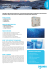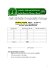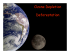# Version A Section

## Transcription

Version A Section
```NAME _____________________________________________ Student ID No. ______________
Section (circle one): A01 (Dr. Lipson)
A02 (Dr. Briggs)
A03 (Dr. Brolo)
UNIVERSITY OF VICTORIA
Version
A
CHEMISTRY 102
Term Test I – February 4, 2011
Version
A
This test has two parts: (A Data Sheet is provided with the exam.)
PART I is a multiple choice examination and is worth 26 marks. The answers for the 14 questions in
this part must be coded on the optical sense form using a soft-leaded pencil.
PART II has written answer questions and is on the last 3 pages and is worth 24 marks. Answer
these questions on this examination paper.
Part II
Score
Exam results
1 [3 marks]
6. [4 marks]
2 [4 marks]
Part I multiple
choice
[26 marks]
Part II written
[24 marks]
Total score
[50 marks]
Percent score
3 [4 marks]
4 [3 marks]
5. [6 marks]
Score
Hand in this entire test paper as well as your optical sense form (bubble sheet) at the
end of the examination period (60 minutes)
PART I – Multiple Choice. Select the best response from the choice provided. Mark only one response for
each question.
1.
This is exam version A. Mark "A" as the answer to question 1
2.
A sample of O2 gas with a volume of 0.357 L was collected at 21oC. Calculate the volume in liters of
the gas when it is cooled to 10oC if the gas is ideal and the pressure remains constant.
A. 0.171
B. 0.290
C. 0.344
D. 0.357
E. 0.371
Chem 102, Midterm 1
Version A page 2 of 7
3. Vessel A contains CO(g) at 0°C and 1 atm. Vessel B contains SO2 (g) at 0 °C and 1 atm. The two
vessels have the same volume. Which of the following statement(s) are TRUE
(i)
Vessel A contains more molecules.
(ii)
Vessel B contains more mass of gas.
(iii) The average kinetic energy of molecules in Vessel B is higher.
(iv)
The root mean square (rms) speed of molecules in Vessel A is higher.
A. (i) & (ii)
4.
D. (ii) & (iv)
E. (i) & (iv)
B. 0.33
C. 0.50
D. 0.67
E. 2.0
Consider the following 5 gases. If an equal mass of each gas is placed into a separate balloon, which gas
sample will have the greatest volume? Assume that the balloons are all at the same temperature and
pressure.
A. He
6.
C. (iii) & (iv)
A container holds a mixture of oxygen, neon and helium gases. The partial pressures are 150 torr for
oxygen, 300 torr for neon and 450 torr for helium. What is the mole fraction for neon?
A. 0.17
5.
B. (i) & (iii)
B. H2
C. N2
D. Ne
E. O2
Consider three samples of gas: N2 at 273 K, H2 at 350 K and O2 at 350 K. Compare the average kinetic
energies of the molecules in these three samples.
A. Additional pressure information is needed to compare average kinetic energies.
B. The heaviest gas (O2) has the highest average kinetic energy and the other average kinetic energies
decrease with molar mass.
C. O2 (350 K) and H2 (350 K) have the same average kinetic energy while N2 (273 K) has a lower
average kinetic energy
D. O2 (350 K) and H2 (350 K) have the same average kinetic energy while N2 (273 K) has a higher
average kinetic energy
E. The lightest gas (H2) has the highest average kinetic energy and the other average kinetic energies
decrease as molar mass increases.
Chem 102, Midterm 1
7.
Version A page 3 of 7
Which one of the following statements about the earth’s atmosphere is CORRECT?
A. The mechanism for restoring the ozone layer over Antarctica involves the formation of polar
stratospheric clouds
B. The first step in the formation of ozone in the stratosphere is a photodissociation reaction.
C. All wavelengths of solar radiation can initiate photoionization reactions.
D. The temperature of the atmosphere steadily decreases with increasing altitude.
E. The ‘hole’ in the ozone layer over Antarctica is of constant ‘depth’ and size throughout the year.
8.
Suppose that the mid-day concentration of NO2 in the ground-level air in a city is 0.020 ppm by volume.
Calculate the number of moles of NO2 in 1.0 L of this air at STP (i.e. at 0 °C and 1 atm).
A. 2.0 x 10-8
9.
B. 8.9 x 10-10
C. 0.45
D. 4.5 x 10-7
E. 0.02
It has been suggested that one way to repair the problem of stratospheric ozone depletion would be to
manufacture ozone, carry it to the stratosphere, and release it. It has been estimated that it would take
about 17 million airplane-loads of ozone to replenish even 10% of the stratospheric ozone. The best
reason(s) that such as solution is impractical is/are:
(i) Even if we could temporarily replace the lost ozone, the natural steady-state ozone
cycle would quickly be re-established at the pre-existing lower ozone concentrations.
(ii) Ozone is too heavy to transport.
(iii) The sudden burst of ozone would cause a climate warming problem.
(iv) Ozone is too reactive to be stored in tanks for transport.
(v) Ozone, being denser than oxygen, would sink to the earth’s surface and cause damage.
A. i
B. iii and iv
D. i and iv
E. i, ii, iii, iv, and v
C. ii and v
10. Which of the following gases are greenhouse gases?
A. O2, H2O
B. CO2, N2
D. CO2, O2, H2O
E. CO2, H2O, CH4
C. N2, H2O
Chem 102, Midterm 1
Version A page 4 of 7
11. In North America, what is the main human-caused source of the sulfur dioxide (SO2) that contributes to
such pollution problems as acid rain?
A. Burning gasoline in cars and trucks.
B. Burning coal and heavy oil for electricity, heating, and industry.
C. Volcanic gases and forest fires.
D. Mining of sulfur.
E. Decomposition of atmospheric sulfur trioxide (SO3).
12. Which of the following statements is NOT CORRECT?
A. Visible and ultraviolet radiation wavelengths reach the earth’s surface and this energy is re-emitted
by the earth as infra-red radiation (heat).
B. Absorption of infra-red radiation increases vibrational energy in a molecule.
C. NO2 in the atmosphere is a significant contributor to the problem of acid rain.
D. CO2, CH4, and water vapour are all greenhouse gases.
E. Unburned hydrocarbons in the stratosphere are a significant contributor to the problem of
photochemical smog.
13. Which of the following reactions is/are NOT a significant process in the usual set of reactions that
maintain the protective stratospheric ozone layer?
(i) O(g) + O2(g) —> O3*(g)
(ii) O2(g) + 2NO(g) —> 2NO2(g)
(iii) O3(g) + hv —> O2(g) + O(g)
A. (i)
D. (i) and (ii)
B. (ii)
C. (iii)
E. (ii) and (iii)
14. A system that does not do work but absorbs heat from the surroundings has
A. q > 0 and ΔE < 0
B. q > 0 and ΔE > 0
C. q < 0 and ΔE > 0
D. q < 0 and ΔE < 0
E. q > 0 and ΔE = 0
Chem 102, Midterm 1
Version A page 5 of 7
(3 marks)
1. Consider the following data about three identical flasks, numbered 1, 2, and 3. Each flask contains a
sample of one gas; one flask contains hydrogen gas (H2), another nitrogen (N2), and a third argon gas
(Ar).
o Each flask contains 4.00 grams of the gas.
o At the same temperature, the pressure in Flask #1 is lower than the pressure in Flask #2.
o In order for the pressure in Flask #3 to be equal to the pressure in Flask #2, Flask #3 must be cooled
to a lower temperature.
Point 1 tells us that the flasks contain different numbers of moles of gases.
Point 2 tells us that there are more moles of gas in flask #2 than in flask #1.
Point 3 tells us that there are more moles of gas in flask #3 than in flask #2.
Therefore,
moles gas in flask #3 > moles gas in flask #2 > moles gas in flask #1
Therefore, flask #3 must contain the lightest gas and flask #1 the heaviest.
(4 marks)
2.
A piece of sodium metal reacts completely with water as follows:
2 NaOH (aq) + H2 (g)
2 Na (s) + 2 H2O (l)
The hydrogen gas generated is collected over water at 23.0°C. The volume of the gas collected is 323
mL measured at 1.010 atm pressure. Calculate the number of grams of sodium metal consumed in the
reaction. (The vapour pressure of water at 23.0 °C is 0.0277 atm.) (There’s extra space on next page.)
P(hydrogen) = 1.010 atm – 0.0277 atm = 0.9823 atm
chemical amount H2 = PV/RT = 0.9823 atm × 323 mL
× 101325 N m-2 atm-1 × 10-6 m3 mL-1
8.314 J mol-1 K-1 × (273.15 + 23.0) K × 1 N m J-1
chemical amount H2 = 0.01306 mol
chemical amount Na used = 2 × 0.01306 mol = 0.02612 mol
mass of Na consumed = 0.02612 mol × 22.99 g mol-1 = 0.600 g (three sig fig)
Chem 102, Midterm 1
Version A page 6 of 7
(4 marks)
3.
Suppose 1000 moles of oxygen gas (O2) is compressed into a steel tank that has a volume of 45.0 L.
(a) Assuming that oxygen behaves as an ideal gas, and that the temperature of the gas and tank is 0°C,
calculate the pressure inside the tank in atmospheres.
P = nRT/V = (1000 mol × 8.314 J mol-1 K-1 × 273.15 K)/ 0.045 m3 = 5.047 × 107 N m-2 (=5.047 × 107 Pa)
converting to atmosphere units
P = 5.047 × 107 N m-2 atm = 498 atm
101325 N m-2
(b) The actual pressure for the real gas in the tank described above is 1026 atm. Explain briefly the
reason for any difference between this value and the result of your calculation in part (a) above.
The real value is higher because molecules take up space, and at high pressures this becomes important.
(3 marks)
4.
Assume that the kinetic energy of a 1400 kg car moving at 115 km h-1 can be converted entirely into
heat by putting on the brakes and bringing the car to a stop.
(a) How much heat (in kilojoules) is that?
Ek = (1/2)mv2 = (1/2) 1400 kg × ((115 × 103 m h-1)/ 3600 s h-1)2 = 700 kg × 1020.4 m2 s-2
Ek (all converted to heat in bringing the car to v=0) = 714313 J = 714 kJ
(b) Suppose that this car is brought to a stop by using its brakes. If we define the system to be the car,
is the internal energy change (ΔE) positive, negative, or zero when the car is brought to a stop using
its brakes? (Hint: The brakes get hot.) Circle the correct answer.
positive
negative
zero
Chem 102, Midterm 1
Version A page 7 of 7
6 marks
5.  a) Write two chemical equations to show the main mechanism of formation of ozone in the
stratospheric ozone layer.
O2(g) + hv  2 O (g)
O (g) + O2(g)  O3*
 b) Write a chemical equation to show the main method of natural destruction of ozone in the stratosphere.
O3(g) + hv  O2(g)
+ O(g)
4 marks
6. Hemoglobin is the protein that transports O2 through the blood from the lungs to the rest of the body. In
doing so each molecule of hemoglobin combines with four molecules of oxygen (O2). The toxicity of the
pollutant gas carbon monoxide (CO) arises because it binds more tightly to hemoglobin than does oxygen.
If 1.00 g of hemoglobin combines with 1.53 × 10-6 m3 of CO at 37oC and 99.0 kPa, what is the molar mass
of hemoglobin?
1 hemoglobin + 4CO  products
for CO, n = PV/RT = 99.0 × 103 Pa × 1.53 × 10-6 m3_______
= 5.874 × 10-5 mol CO
8.314 J mol-1 K-1 × (273.15 + 37) K
moles hemoglobin combined = (1/4) × 5.874 × 10-5 mol = 1.469 × 10-5 mol
molar mass hemoglobin = (mass)/(moles) = 1.00g/1.469 × 10-5mol
= 68096 g/mol = 6.81 × 104 g/mol
~~~~~~~~~~~~~~~~~~~~~~~~~~~~~~~~~~~~END~~~~~~~~~~~~~~~~~~~~~~~~~~~~~~~~~~~~
```

### Ultimate Gas Laws Worksheet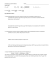### Ozone Sparging System - Sirco Industrial, Inc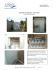### Flyer for AAOT meeting 2013 copy 6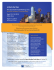### 251. The Fleaker - UC DRC Home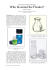### Document 6529186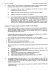### ThE ProzoNE TwistEr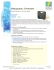### Louise Irvine, the renowned Royal Doulton expert and author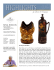### Version A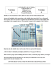### OZAT® CFS